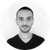# New to Qlik Sense

If you’re new to Qlik Sense, start with this Discussion Board and get up-to-speed quickly.

cancel
Showing results for
Did you mean:Not applicable

## If statement combined with set analysis

Hi everyone!

Im new to qlik sense and having problems with combining set analysis with an if statement. I am making a line chart and only want the last twelve months to be shown, but I also want a filter on a dimension. Its when Im adding the if statement that it stops working.

CustomerGroup=Numeric dimension

Indicator=Sum(NBCustomer)

if(CustomerGroup=1, Sum({\$<Month=, Year=, Date={">=\$(=MonthStart(AddMonths(Max(Date),-12)))<\$(=MonthEnd(Max(Date)))"}>} [NbCustomer]))

This part works seperatly:

Sum({\$<Month=, Year=, Date={">=\$(=MonthStart(AddMonths(Max(Date),-12)))<\$(=MonthEnd(Max(Date)))"}>} [NbCustomer])

Please help!!Kind regards,

Sofia

1 Solution

Accepted SolutionsLuminary

Try this for your expression:

Sum({\$<CustomerGroup={'1'}, Month=, Year=, Date={">=\$(=MonthStart(AddMonths(Max(Date),-12)))<\$(=MonthEnd(Max(Date)))"}>} [NbCustomer])

vizmind.eu
8 RepliesPartner - Master III

please tell us which dimensions and measures you are using in line chart.Not applicable
Author

Hello!

CustomerGroup=Numeric dimension

Indicator=Sum(NBCustomer)

Date=date dimensionPartner - Master III

why you need to use IF ?Not applicable
Author

I probably dont! If you have a better way I would very much appreciate it!Kind regards,

SofiaLuminary

Try this for your expression:

Sum({\$<CustomerGroup={'1'}, Month=, Year=, Date={">=\$(=MonthStart(AddMonths(Max(Date),-12)))<\$(=MonthEnd(Max(Date)))"}>} [NbCustomer])

vizmind.euEmployee

Sum({\$<CustomerGroup*={"1"},Month=, Year=, Date={">=\$(=MonthStart(AddMonths(Max(Date),-12)))<\$(=MonthEnd(Max(Date)))"}>} [NbCustomer])Partner - Master III

you can use set analysis also for CustomerGroup like below:

Sum({\$<Month=, Year=, Date={">=\$(=MonthStart(AddMonths(Max(Date),-12)))<\$(=MonthEnd(Max(Date)))"}, CustomerGroup = {"1"} >} [NbCustomer])

this will only filter CustomerGroup  = 1 regardless which CustomerGroup you selected, else you can use CustomerGroup *= {"1"} to take care also of CustomerGroup selections.Not applicable
Author

Thank you everyone! Now it works.

Kind regards,

SofiaTags
Community Browser